# How to simplify ratios with fractions

Want to find out how to simplify ratios with fractions? We've provided a step-by-step guide of how to simplify ratios with fractions to teach you everything you need to know.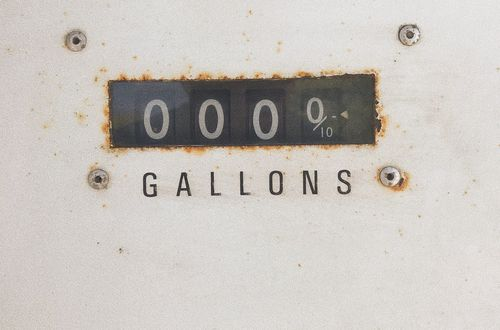## Step 1: Convert to improper fractions

Check for any mixed fractions and change them to improper fractions first. If there are no mixed fractions, proceed to Step 2 right away. Given a ratio with fractions such as 1 5/12 ∶7/30 , we can first change the mixed fraction to an improper fraction.

## Step 2: Find the least common denominators

Find the least common multiple of the denominators. Now that we have 17/12 ∶7/30 , we determine the LCD shared by the two fractions.

## Step 3: Multiply the least common denominators

Multiply the LCD on the values of the given ratio. This means that for our example, we multiply 60 to both 17/12 and 7/30 .

## Step 4: Express as a simplified ratio

Express the product as the new ratio. Simplify the ratio whenever possible. Hence, we have:

## Examples of how to simplify ratios with fractions

**Q1) Simplify the ratio 12/17 ∶15/68. **

We first determine the least common denominator (LCD) shared by 17 and 68. 17=17×1 68=17×4 LCD=17×4=68

Multiply the LCD to the values in the ratio so that we end up with whole numbers on both sides.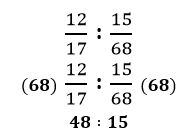Once we have the products as a whole number, we can now cancel common factors out to further simplify the new ratio. 48÷3:15÷3 =16:5

Hence, we have: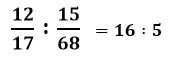**Q2) Simplify the ratio 3 2/9 ∶5/18 ∶11/24 to its lowest term. **

We first change 3 2/9 to an improper fraction.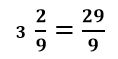Rewriting the ratio with the improper fraction, we have: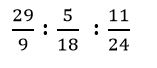Let’s now look for the least common denominator shared by the fractions. 9=3×3 18=3×3×2 24=3×2×2×2 LCD=3×3×2×2×2=72

Multiply 72 on all three fractions. Simplify when needed.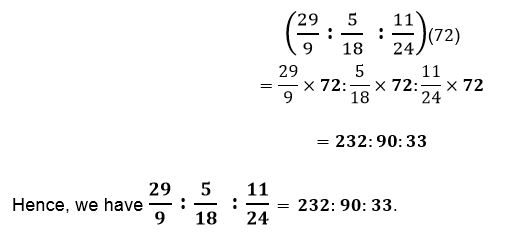#### Join today

The fastest way to practice

Unlock our complete testing platform and improve faster that ever.